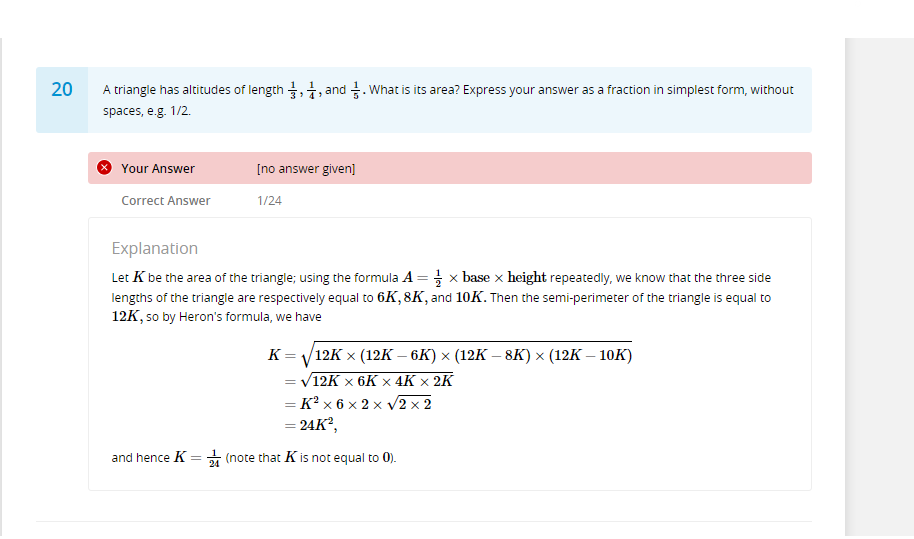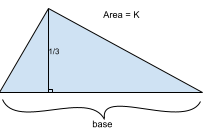# q's comes from module 2 week 4 challenge Q#20

• here is a question that relates to calculating a triangle's area when the question has already given you the three sides. My question is why choose 6K, 8K, and 10K? Why not use the original three numbers - 3K,4K, and 5K?• Hey @victorioussheep !

So the area of the triangle is $$K$$, and we know this is equal to $$\frac12 \cdot \text{(base)} \cdot \text{(height)}$$. Keep in mind that altitude and height are the same thing, and the problem actually gives the values for the altitudes!

Since one of the altitudes is $$\frac13$$, we can say that

$$K=\frac12 \cdot \text{(base)} \cdot \frac13$$
$$3K=\frac12 \cdot \text{(base)}$$
$$6K=\text{(base)}$$

So, the length of the base that has altitude $$\frac13$$ is equal to $$6K$$.

We can actually do the same thing for the other two bases!

Base with altitude $$\frac14$$:
$$K=\frac12 \cdot \text{(base)} \cdot \frac14$$
$$8K=\text{(base)}$$

Base with altitude $$\frac15$$:
$$K=\frac12 \cdot \text{(base)} \cdot \frac15$$
$$10K=\text{(base)}$$

That means the three bases (which are the sides) of the triangle are $$6K, 8K,$$ and $$10K$$! Hope this cleared up the confusion• @quacker88
okkkk..... so they basically use 6K, 8K, and 10K because they are the whole numbers so it's convenient to use?

• @victorioussheep not quite -- the numbers were actually calculated.

Remember, $$K$$ is the variable we set for the area. The goal of the problem is to find $$K$$. But here's the thing, we need the side lengths of the triangle to find the area, right? So, the calculations I did earlier were to find the side lengths of the triangle.

Remember, $$\textbf{(area)} = \frac12 \cdot \textbf{(base)} \cdot \textbf{(height)}$$, right? (that rhymes haha)

Well, we set the area as $$K$$ earlier, and the problem says that the length of one of the altitudes (the height) is equal to $$\frac13$$. Here's a (very not to scale) sketch of what we have so far:Well, we know the area and we know the height, so let's solve for the base!

$$\text{(area)} = \frac12 \cdot \text{(base)} \cdot \text{(height)}$$
$$K=\frac12 \cdot \text{(base)} \cdot \frac13$$
$$6K=\text{(base)}$$

So the length of the base is $$6K$$. Remember, we don't know exactly what $$K$$ is yet (it's what we're solving for!) but we do know that the base is equal to $$6K$$.

You can do this process for all three sides and you'll get that the side lengths are $$6K, 8K,$$ and $$10K$$! Does this make a little more sense now?

• @quacker88
oh yes! it definitely makes more sense now! I really like how you use visuals in between your explanation! It makes me understand the concept a lot quicker, and thanks again!

• @victorioussheep Glad that it helped!